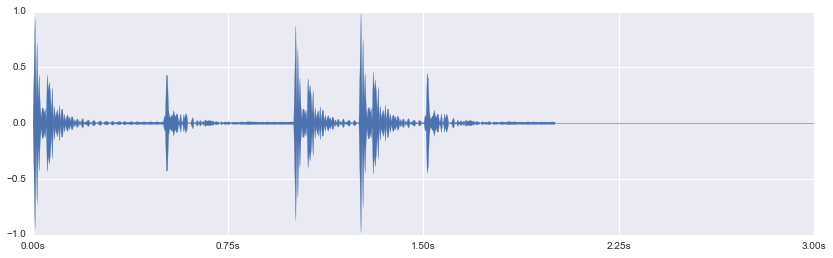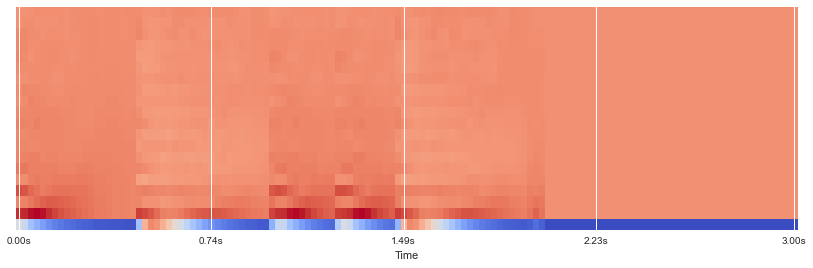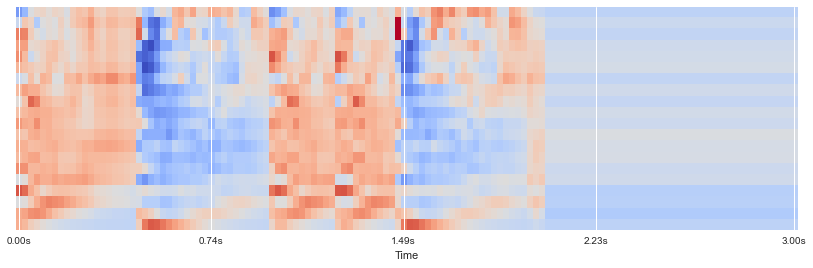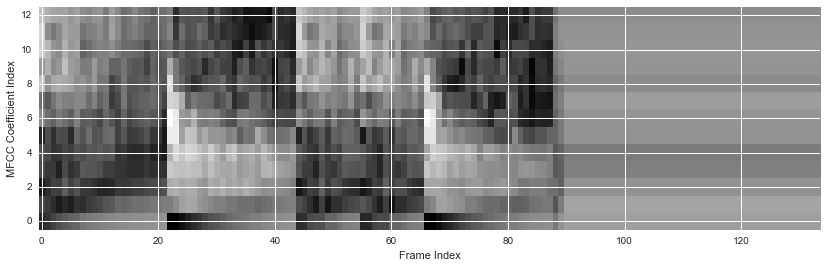In :
import numpy, scipy, matplotlib.pyplot as plt, sklearn, librosa, urllib, IPython.display
import essentia, essentia.standard as ess
plt.rcParams['figure.figsize'] = (14,4)


# Mel Frequency Cepstral Coefficients (MFCCs)¶

The mel frequency cepstral coefficients (MFCCs) of a signal are a small set of features (usually about 10-20) which concisely describe the overall shape of a spectral envelope. In MIR, it is often used to describe timbre.

In :
url = 'http://audio.musicinformationretrieval.com/simple_loop.wav'
urllib.urlretrieve(url, filename='simple_loop.wav')

Out:
('simple_loop.wav', <httplib.HTTPMessage instance at 0x10ec5cd88>)

Plot the audio signal:

In :
x, fs = librosa.load('simple_loop.wav')
librosa.display.waveplot(x, sr=fs)

Out:
<matplotlib.collections.PolyCollection at 0x10ecb5fd0>Play the audio:

In :
IPython.display.Audio(x, rate=fs)

Out:

## librosa.feature.mfcc¶

librosa.feature.mfcc computes MFCCs across an audio signal:

In :
mfccs = librosa.feature.mfcc(x, sr=fs)
print mfccs.shape

(20, 130)


In this case, mfcc computed 20 MFCCs over 130 frames.

The very first MFCC, the 0th coefficient, does not convey information relevant to the overall shape of the spectrum. It only conveys a constant offset, i.e. adding a constant value to the entire spectrum. Therefore, many practitioners will discard the first MFCC when performing classification. For now, we will use the MFCCs as is.

Display the MFCCs:

In :
librosa.display.specshow(mfccs, sr=fs, x_axis='time')

Out:
<matplotlib.image.AxesImage at 0x106318c50>### Feature Scaling¶

Let's scale the MFCCs such that each coefficient dimension has zero mean and unit variance:

In :
mfccs = sklearn.preprocessing.scale(mfccs, axis=1)
print mfccs.mean(axis=1)
print mfccs.var(axis=1)

[ -4.64585635e-16  -1.63971401e-16  -1.09314267e-16  -1.09314267e-16
0.00000000e+00   1.09314267e-16   0.00000000e+00  -1.09314267e-16
-1.09314267e-16  -2.73285668e-17   1.09314267e-16  -8.19857003e-17
5.46571335e-17   0.00000000e+00   2.73285668e-17  -4.09928501e-17
1.09314267e-16   8.19857003e-17   9.56499837e-17   6.83214169e-17]
[ 1.  1.  1.  1.  1.  1.  1.  1.  1.  1.  1.  1.  1.  1.  1.  1.  1.  1.
1.  1.]


Display the scaled MFCCs:

In :
librosa.display.specshow(mfccs, sr=fs, x_axis='time')

Out:
<matplotlib.image.AxesImage at 0x1108b8890>## essentia.standard.MFCC¶

We can also use essentia.standard.MFCC to compute MFCCs across a signal, and we will display them as a "MFCC-gram":

In :
hamming_window = ess.Windowing(type='hamming')
spectrum = ess.Spectrum()  # we just want the magnitude spectrum
mfcc = ess.MFCC(numberCoefficients=13)
frame_sz = 1024
hop_sz = 500

mfccs = numpy.array([mfcc(spectrum(hamming_window(frame)))
for frame in ess.FrameGenerator(x, frameSize=frame_sz, hopSize=hop_sz)])
print mfccs.shape

(134, 13)


Scale the MFCCs:

In :
mfccs = sklearn.preprocessing.scale(mfccs)

/usr/local/lib/python2.7/site-packages/sklearn/preprocessing/data.py:153: UserWarning: Numerical issues were encountered when centering the data and might not be solved. Dataset may contain too large values. You may need to prescale your features.
warnings.warn("Numerical issues were encountered "
/usr/local/lib/python2.7/site-packages/sklearn/preprocessing/data.py:169: UserWarning: Numerical issues were encountered when scaling the data and might not be solved. The standard deviation of the data is probably very close to 0.
warnings.warn("Numerical issues were encountered "


Plot the MFCCs:

In :
plt.imshow(mfccs.T, origin='lower', aspect='auto', interpolation='nearest')
plt.ylabel('MFCC Coefficient Index')
plt.xlabel('Frame Index')

Out:
<matplotlib.text.Text at 0x1108cde10>